Types of matrices

Chapter 3 Class 12 Matrices
Concept wise

Let’s learn the different types of matrices

## Column matrices

A column matrix only has 1 column

Example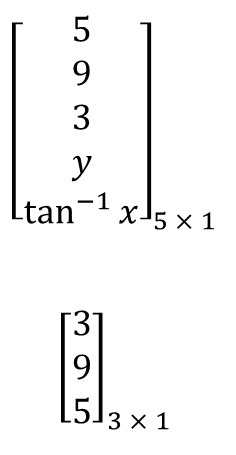Example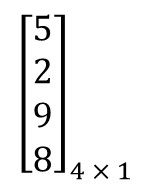So, column matrix is of the order m × 1

We write it as

A = [a ij ] m × 1

## Row matrices

A row matrix only has 1 row

Example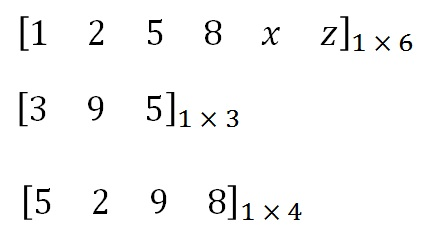So, row matrix is of the order 1 × n

We write it as

A = [a ij ] 1 × n

## Square matrix

A square matrix has equal number of rows & columns

Example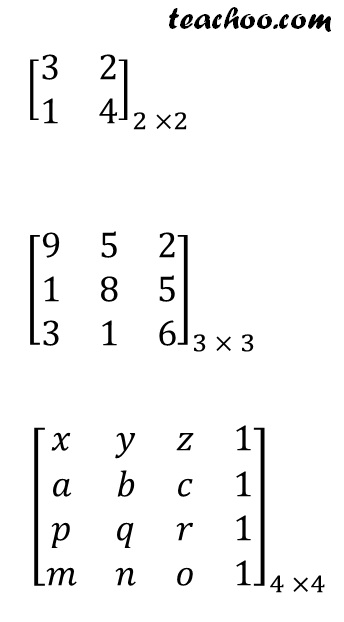So, a square matrix is of the order m × m

We write it as

A = [a ij ] m × m

## Diagonal matrix

In A diagonal matrix, the non-diagonal of element are zero.

A diagonal matrix is possible only in a square matrix

Example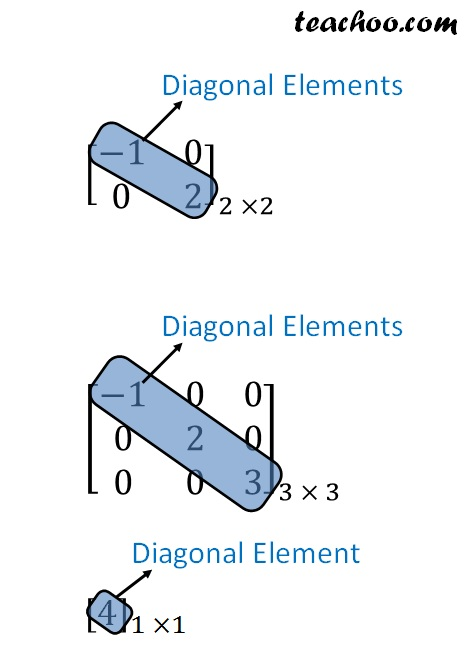So, in a diagonal matrix

• It is should be a square matrix
• Non-diagonal elements are 0

## Scalar matrix

A scalar matrix is a diagonal matrix where diagonal elements are equal

Example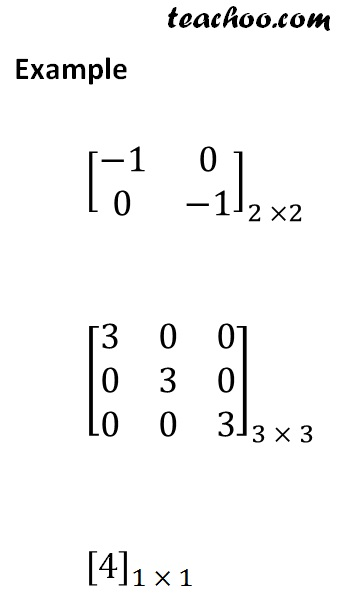So, in a scalar matrix

• It is a square matrix
• Non diagonal elements are 0
• Diagonal elements are equal

## Identity matrix

An identity matrix is a diagonal matrix where all diagonal elements are 1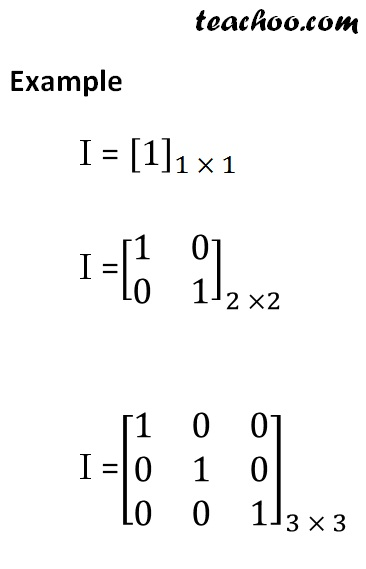So, in a Identity matrix

• It is a square matrix
• Non diagonal elements are 0
• All diagonal elements are 1

Note : An identity matrix is a scalar matrix

Learn in your speed, with individual attention - Teachoo Maths 1-on-1 Class

### Transcript

[■8(5@9@3@𝑦@tan^(−1)⁡𝑥 )]_(5 × 1) [■8(3@9@5)]_(3 × 1) [■8(5@2@9@8)]_(4 × 1) [■8(3&2@1&4)]_(2 ×2) [■8(9&5&2@1&8&5@3&1&6)]_(3 × 3) [■8(𝑥&𝑦&𝑧&1@𝑎&𝑏&𝑐&1@𝑝&𝑞&𝑟&1@𝑚&𝑛&𝑜&1)]_(4 ×4) Example Diagonal Elements Diagonal Elements [■8(−1&0&0@0&2&0@0&0&3)]_(3 × 3) Diagonal Element _(1 ×1) [■8(−1&0@0&−1)]_(2 ×2) [■8(3&0&0@0&3&0@0&0&3)]_(3 × 3) _(1 × 1) I = _(1 × 1) I =[■8(1&0@0&1)]_(2 ×2) I =[■8(1&0&0@0&1&0@0&0&1)]_(3 × 3)